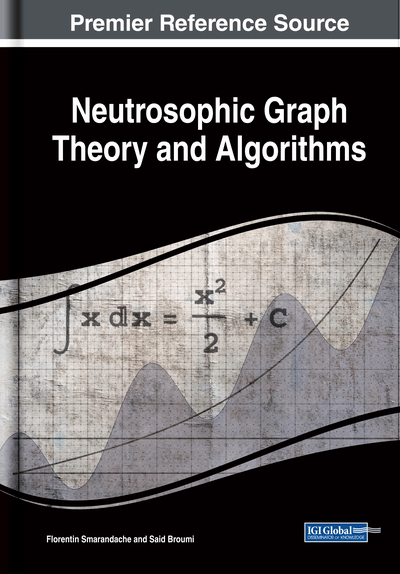# Neutrosophic Soft Digraph

Kalyan Sinha (Department of Mathematics, A.B.N. Seal College, India) and Pinaki Majumdar (Department of Mathematics, MUC Woman's College, India)
DOI: 10.4018/978-1-7998-1313-2.ch012
Available
\$37.50
No Current Special Offers

## Abstract

Neutrosophic soft sets are an important tool to deal with the uncertainty-based real and scientific problems. In this chapter, the idea of neutrosophic soft (NS) digraph has been developed. These digraphs are mainly the graphical representation of neutrosophic soft sets. A graphical study of various set theoretic operations such as union, intersection, complement, cross product, etc. are shown here. Also, some properties of NS digraphs along with theoretical concepts are shown here. In the last part of the chapter, a decision-making problem has been solved with the help of NS digraphs. Also, an algorithm is provided to solve the decision-making problems using NS digraph. Finally, a comparative study with proposed future work along this direction has been provided.
Chapter Preview
Top

## 1. Introduction

Prof. (L. A. Zadeh, 1965) introduced the concept of fuzzy sets in 1965. Soft Set theory was introduced by (Molodtsov, 1999) as a parametric tool to deal the uncertain data which is present in many mathematical problems. In his paper, several applications of this theory are shown by him. Also (Maji et al., 2003; 2004) have further studied the theory of soft sets and the concept of fuzzy soft set and intuitionistic fuzzy soft set, vague set (Maji et al., 2001; 2001a; 2002; 2003a; 2004, Gau et al., 1993), a more generalized concept was introduced by them. Gradually research in soft set theory (SST) are grown up in many areas like algebra, entropy calculation, applications (see Atanasov, 1986; R. Kumar et al., 2018; 2019a; 2019b; 2019c; P.K. Maji, 2013), for example).

On the other hand based on neutrosophic logic and sets, a new theory has been developed. (Prof F. Smarandache, 2006) invented the theory of Neutrosophic Set (NS) in which every set has truth member ship (T), indeterminacy membership (I) and falsity membership (F).

In neutrosophic sets, all the membership degree lie in ] 0, 1[. Unlike in IFS in which uncertainty is a function of the degree of belongingness as well as degree of non-belongingness, here the indeterminacy factor is present in the form of uncertainty. Clearly truth and falsity values is not a function of indeterminacy. Works on soft sets and neutrosophic sets are progressing very rapidly (Ali et al., 2016; 2017; Ansari et al., 2013; Aydodu, 2015; Broumi et al., 2014, 2016a, Smarandache, 2011, 2015c). In 2013, (P.K. Maji, 2013) gave the idea of Neutrosophic Soft Set theory.

In current years, mathematicians use graph theory in large scale to solve many open problems in modern mathematics. As a consequence, research in fuzzy graph theory, fuzzy digraph theory, intuitionistic fuzzy graphs, soft digraphs (Broumi et al., 2019; Gani et al., 2003; 2010; 2012; Karaaslan, 2015a; Majumdar et al., 2008; 2010a; 2013b; Majumdar, 2015b; Rosenfield, 1975; Sinha et al., 2016b; 2018a; 2019d) are being carried out very rapidly. However (Samarandache; 2015c, Broumi et al., 2014, 2016a) introduced the concept of Neutrosophic graphs firstly in their papers.

## Complete Chapter List

Search this Book:
Reset